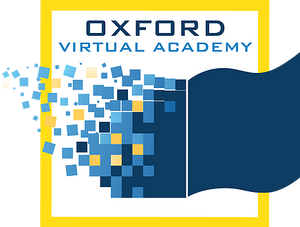# Geometry CR

Geometry is the beginning of creating a solid foundation in mathematics by studying and exploring a wide range of geometric concepts. Students begin to understand the basics of geometric equations and how these equations are present in daily life. Students learn to calculate perimeter and work directly with angles and arcs to understand the importance of geometric math in construction.

* Prerequisites: Algebra I